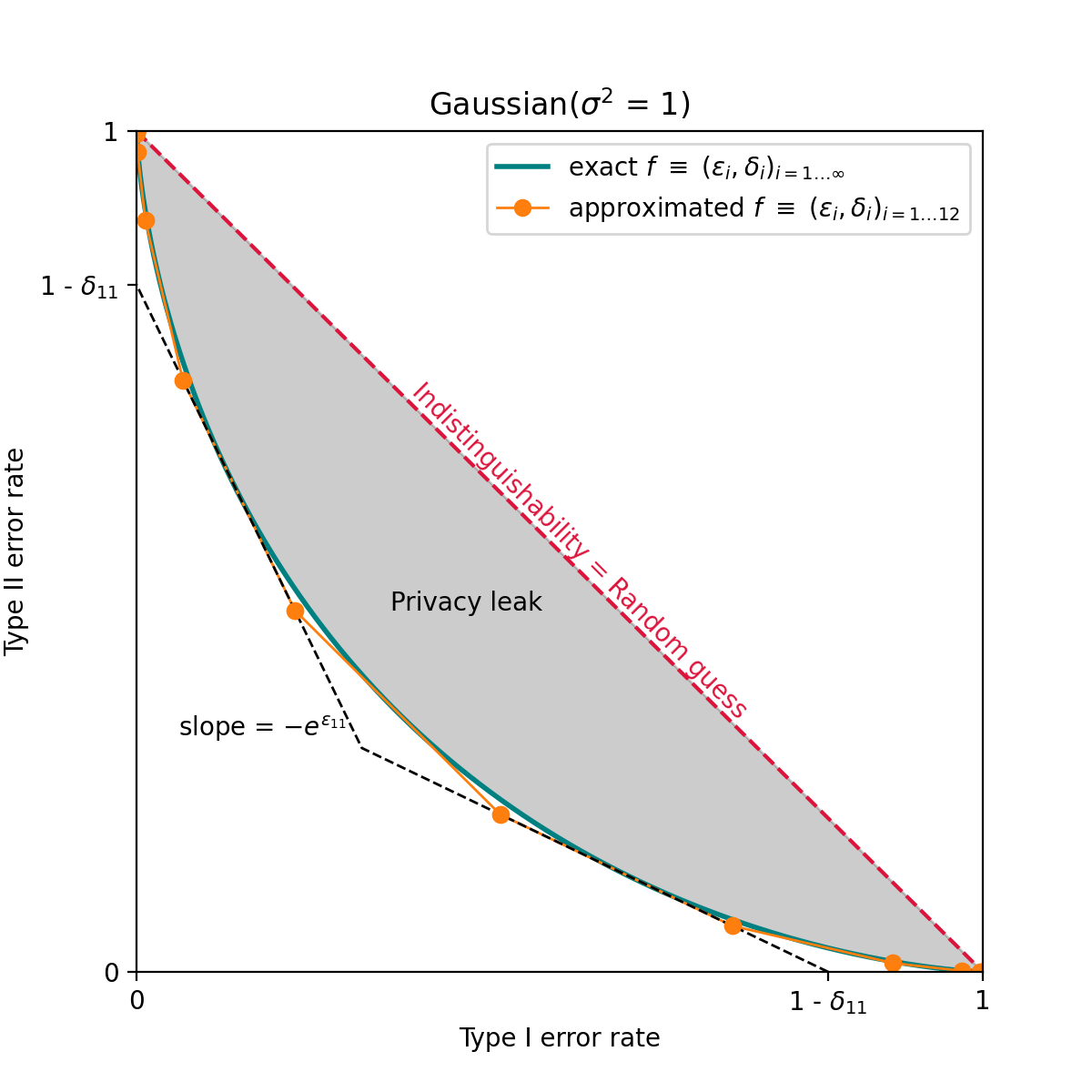Future of differential privacy

# Sarus implements 𝑓-differential privacy in OpenDP

An initiative sponsored by Harvard University

Differential Privacy
Open Source
Victoria de Sainte AgatheTo solve real life data science problems on privacy sensitive data, Sarus Technologies needs to compose large numbers of differentially private mechanisms of all kinds. Keeping a fine privacy accounting of all the various mechanisms is essential to provide formal privacy guarantees. However, this task quickly becomes unmanageable as existing accountants are only efficient for a narrow range of mechanisms leading to suboptimal composition at best or, in the worst case, a breach of privacy guarantees.

A recent framework, 𝑓-differential privacy, appears to address composition of a wide variety of mechanisms. This stems from the fact that it exactly characterizes the privacy of any mechanism and provides an exact formula for composition. Observing that there was no open-source implementation of such a universal accountant, we decided to build one as a contribution to the OpenDP open source library.

## Privacy accounting for real-life data science

Using Sarus software an analyst can access synthetic data, run SQL queries, and train Machine-Learning (ML) models with the gold standard of privacy: Differential Privacy (DP).

Differential privacy is a theoretical framework which allows to account for and set some limits on privacy loss every time one accesses some private data. The kind of mechanisms, and therefore privacy loss profiles, varies depending on the query:

• Sampled Gaussian Mechanisms for deep-learning training using DP-SGD (Abadi et al. 2016, Mironov et al., 2019),
• Composed Exponential and Laplace Mechanisms for other ML applications such as private-boosted-trees (Li et al., 2020),
• Composed Laplace Mechanisms and (𝜀, 𝛿)-differentially private queries with tight 𝛿 for SQL queries (the 𝜏-thresholding mechanism in Wilson et al., 2019).

The variety of queries and private mechanisms makes a comprehensive approach to accounting rather difficult. An accountant based on approximate differential privacy and its generic composition theorems may largely overestimate the privacy consumption. This is the case in particular for the Gaussian mechanism, and it gets worse as the number of compositions increases. Thus a lot of efforts have been put into privacy accounting in the context of DP-SGD (Moment accountant, Concentrated-DP, Rényi-DP). Unfortunately, these divergence based methods which nicely handle the composition of sampled Gaussian mechanisms, do not accurately represent privacy loss across all mechanisms as demonstrated in proposition B.7 of Dong et al., 2019.

## The need for a universal privacy accountant

There are various open-source libraries providing reference implementations for DP mechanisms. Some implementations focus on the cases of DP-SGD (e.g.: Tensorflow privacy), and others on generic mechanisms (OpenDP / Smartnoise, Google differential privacy, IBM/differential-privacy-library), but — as shown below — no implemented accountant seems to allow for an accurate representation of DP-mechanisms across the board.The privacy loss of a mechanism (here the Gaussian mechanism) can be exactly characterized by its tradeoff function. The tradeoff function measure the distance between the probability distributions of the output when the mechanism is applied on two adjacent datasets. The exact tradeoff function (in green) is equivalent to an infinite number of (𝜀, 𝛿) pairs. By selecting only a subset of the (𝜀, 𝛿) over the whole collection, we can reduce the complexity and approximate the exact tradeoff function by a piecewise function (in orange). In approximate differential privacy only one (𝜀, 𝛿) pair is chosen leading to a large overestimating of the privacy consumption.

Recent works from Dong et al. (2019), building upon the seminal work of Kairouz et al. (2015), give a very general and elegant framework to account for privacy: 𝑓-differential privacy. The idea is to exactly characterize the privacy loss of a mechanism by the tradeoff function between the probability distribution of the outputs of the mechanism applied on two adjacent datasets. This is equivalent to a potentially infinite collection of (𝜀, 𝛿) guarantees. The mathematical properties of the tradeoff function ensure that we can then safely (i.e. without underestimating the privacy loss) reduce the complexity by considering only a subset of the whole (𝜀, 𝛿) family.

Observing that there was no open-source implementation of such an accountant, we decided to implement one for the community with the high standards of a peer-reviewed library such as OpenDP.

## Validation of an 𝑓-DP accountant

OpenDP is a community effort led by Harvard University to develop an open source software for analysing sensitive data with vetted differential privacy guarantees. One of the greatest strengths with the OpenDP library is its rigorous contribution process. Each contribution must be peer-reviewed and the contributor has to provide the mathematical proof that his implementation is indeed differentially private.

Our interactions with the OpenDP team allowed us to formalize the idea of using an approximate version of 𝑓-DP. We have submitted two contributions:

• In this version, we have implemented the bijective mappings between the collection of (𝜀, 𝛿), the tradeoff function and the probability distributions of the outputs of the mechanism applied on two adjacent datasets. We switch from one representation to another depending on the use case (e.g.: probability distributions for the composition, (𝜀, 𝛿) for the interaction with the user).
• In this second version, we give a similar implementation where we work mostly with the concept of probability loss distribution described in Sommer et al. (2020). Also, the integration with OpenDP is somewhat different.

In both implementations we use only rational numbers so each operation is exact:

• preventing accidental underestimation of privacy loss due to uncontrolled roundings,
• and avoiding vulnerabilities based on irregular discretization of floats (Mironov, 2012)

Overall, we are super happy to bring practical 𝑓-DP to the community 🥳. We learned a lot on the way. It helped us in particular to validate the design choices of the privacy accountant that will fuel Sarus products.Get in touch, you'll be up and running in no time.
main.py
1
2
3
4
5
6

Shell